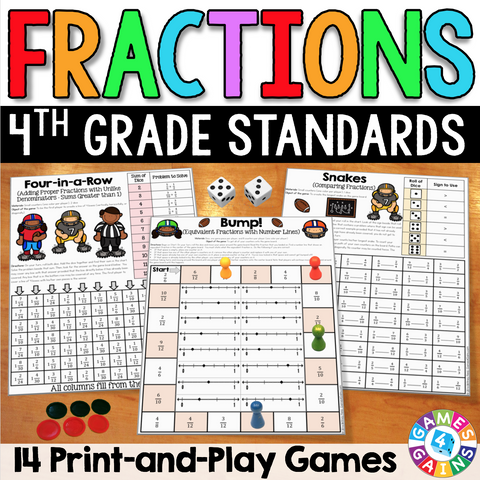## Fractions Games for 4th Grade

• \$500

Fractions Games for 4th Grade contains 14 fun and engaging printable board games to help students to practice many of the 4th grade fractions standards. This pack includes differentiated games for practicing equivalent fractions, comparing fractions, adding fractions, subtracting fractions, and multiplying fractions by whole numbers.

These games are so simple to use and require very minimal prep. They are perfect to use in math centers or as extension activities when students complete their work!

Included with this Fractions Games for 4th Grade set:
• Instructions for play
• 14 printable games

These Fractions Games for 4th Grade support the following 4th Grade Math Common Core standards:

CCSS.MATH.CONTENT.4.NF.A.1
Explain why a fraction a/b is equivalent to a fraction (n × a)/(n × b) by using visual fraction models. Use this principle to recognize and generate equivalent fractions.

CCSS.MATH.CONTENT.4.NF.A.2
Compare two fractions with different numerators and different denominators. Record the results of comparisons with symbols >, =, or <.

CCSS.MATH.CONTENT.4.NF.B.3
Understand a fraction a/b with a > 1 as a sum of fractions 1/b.

CCSS.MATH.CONTENT.4.NF.B.3.A
Understand addition and subtraction of fractions as joining and separating parts referring to the same whole.

CCSS.MATH.CONTENT.4.NF.B.3.B
Decompose a fraction into a sum of fractions with the same denominator in more than one way.

CCSS.MATH.CONTENT.4.NF.B.3.C
Add and subtract mixed numbers with like denominators.

CCSS.MATH.CONTENT.4.NF.B.4
Apply and extend previous understandings of multiplication to multiply a fraction by a whole number.

************

You may also enjoy these other games by Games 4 Gains: